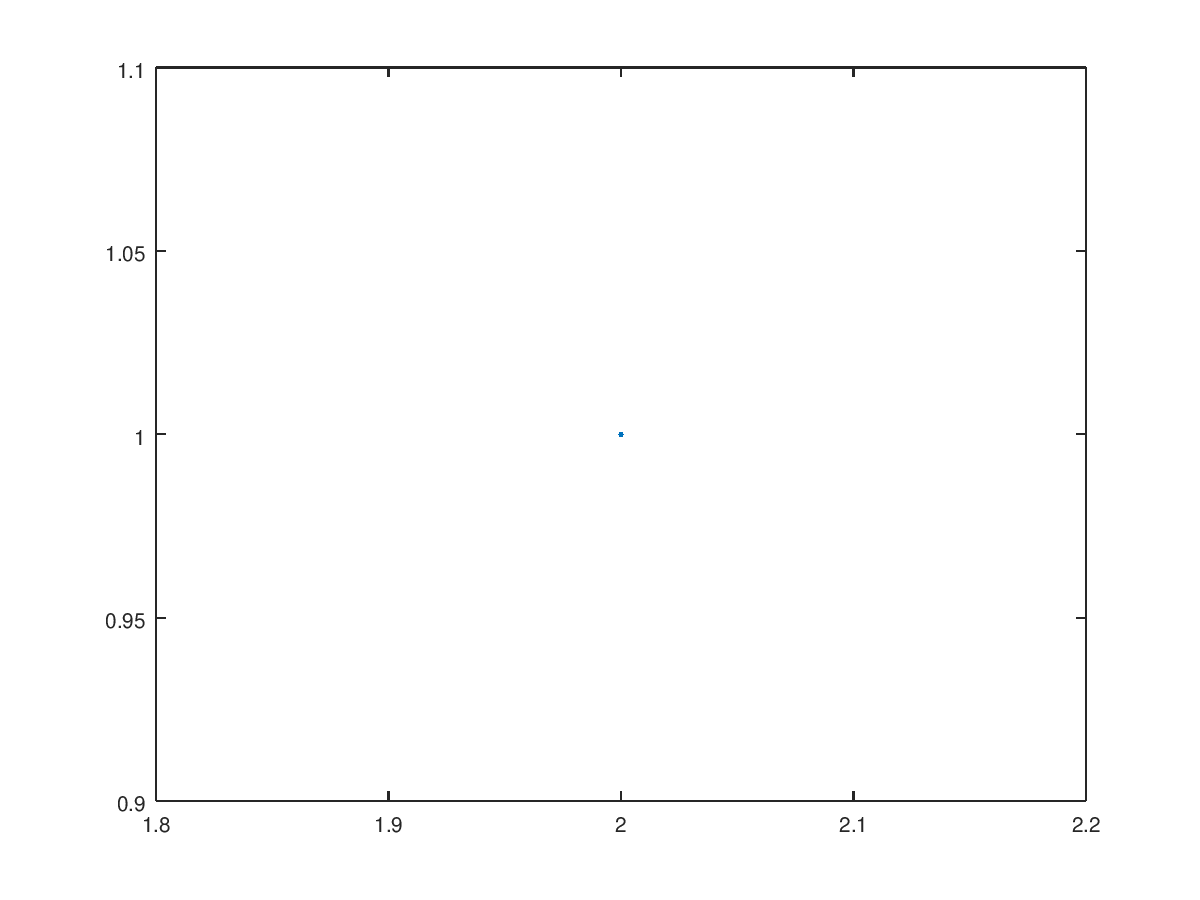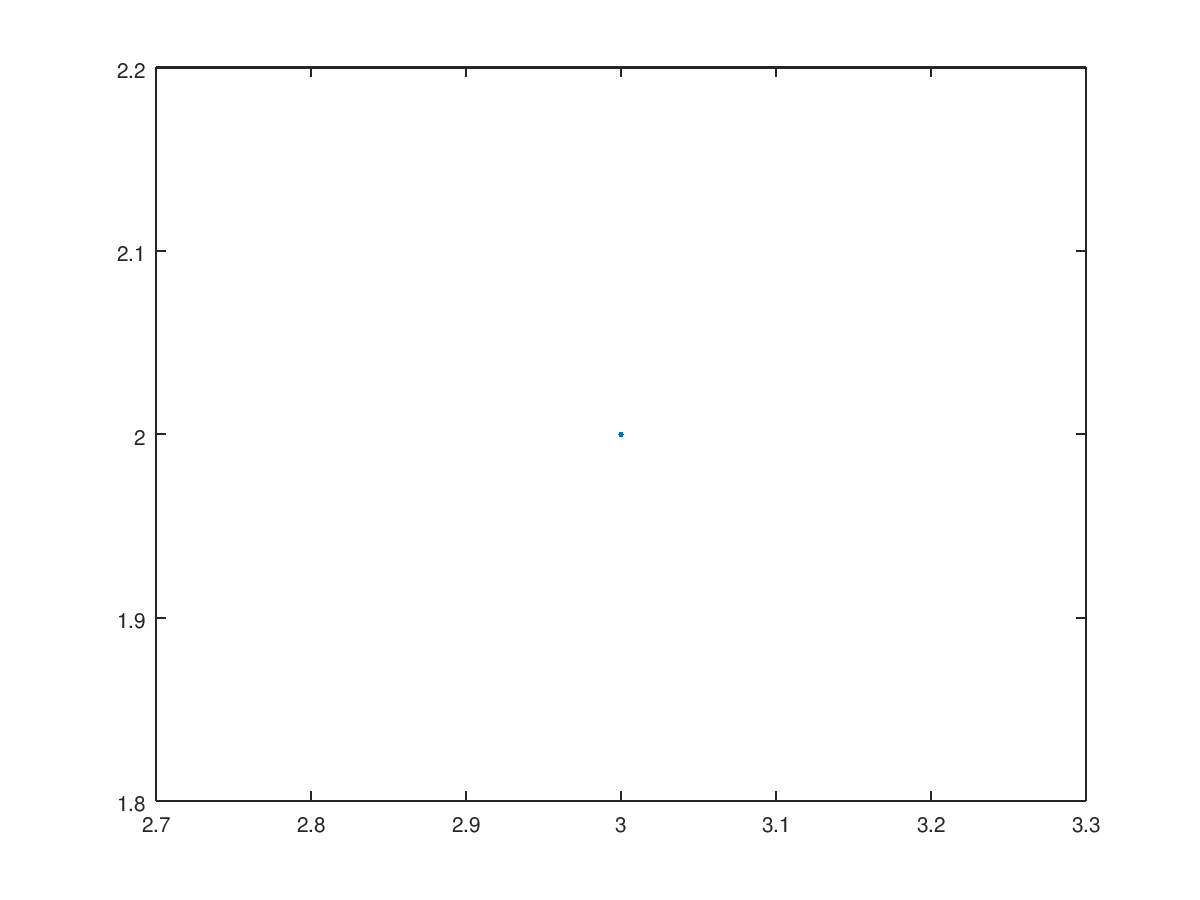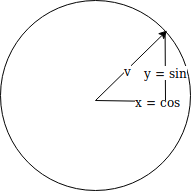# IntroductionWhen we playing we see moving objects around the scenery, so today let's have a look how one of the most important pieces of that movement works: translation. There are many ways to perform the same maths, but the GPU must follow the defined pipeline and that's way we use matrices.

# Translation matrix

First of all we have to think in a single point, a single point can be defined in the following way: p(x, y) where x means its position in abscissas and y it position in coordinates, so let's plot the following point p(2,1),Really simple, right. What happens if we want to move the point in one in both axis, we have to add p(1, 1) to the previous point and the plot result is this:All of this seems to be right, so we move the points by adding values to our current points p'(x, y) = p(x, y) +p(<x movement>, <y movement>). The translation matrix for a point 3D is the following:In this way in our code we just need to add a Matrix4f, that we call World, where each point will be processed:

    Matrix4f World;
World.m = 1.0f; World.m = 0.0f; World.m = 0.0f; World.m = sinf(scale);
World.m = 0.0f; World.m = 1.0f; World.m = 0.0f; World.m = cosf(scale);
World.m = 0.0f; World.m = 0.0f; World.m = 1.0f; World.m = 0.0f;
World.m = 0.0f; World.m = 0.0f; World.m = 0.0f; World.m = 1.0f;

Take a moment to understand the matrix, according to the previous definition we are gonna change the value of Vx and Vy by processing its sin and cos respectively. Vz is always zero. Remember that cos(0) = 1 and sin (0) = 0 so what we se when we run this is that the triangle is following a circumference on its translation movement.

# Rotation matrix

Now what we have is two vectors, one pointing to v = {0, 2} and the second to v' = {2, 0}. We've rotated the first vector by 90 degrees clockwise in order to get the second one, but how to do that? Well, we know tan(α) = sin (α) / cos (α), it's a trigonometry identity, so also the following is true:where α is the angle between the abscissa and the vector v, so we can say that tan(α) = y / x. If we know 'α which is the new angle, somewhing true, we are able to calculate x and y by calculating sin('α) and cos('α), Finally, the rotation matrix is as follows:when applying the rotation on the XY plane. Our translation + rotation matrix is the following:

    World.m = cosf(scale); World.m = -sinf(scale); World.m = 0.0f; World.m = sinf(scale);
World.m = sinf(scale); World.m = cosf(scale);  World.m = 0.0f; World.m = cosf(scale);
World.m = 0.0f;        World.m = 0.0f;         World.m = 1.0f; World.m = 0.0f;
World.m = 0.0f;        World.m = 0.0f;         World.m = 0.0f; World.m = 1.0f;


All code is available here.